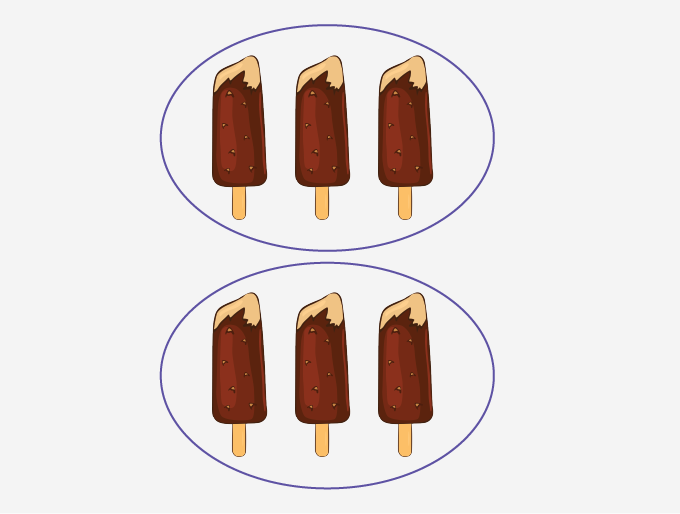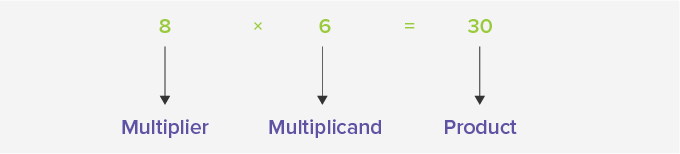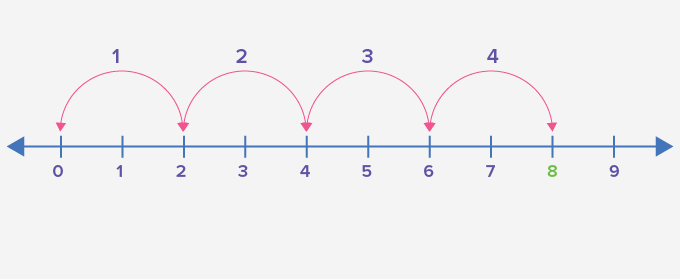# Multiplication - Definition with Examples

The Complete K-5 Math Learning Program Built for Your Child

• 40 Million Kids

Loved by kids and parent worldwide

• 50,000 Schools

Trusted by teachers across schools

• Comprehensive Curriculum

Aligned to Common Core

## What is Multiplication?

Multiplication, one of the four basic operations of arithmetic, gives the result of combining groups of equal sizes.Here each group has 3 ice creams, and there are two such groups. So, there are 2 times 3 or 3 + 3 or 6 ice creams in total. In other words, multiplication is repeated addition.

Multiplication is represented by the signs cross ‘×’, asterisk ‘*’ or dot ‘·’. When we multiply two numbers, the answer we get is called ‘product’. The number of objects in each group is called ‘multiplicand,’ and the number of such equal groups is called ‘multiplier’.

For example:

3 × 7 = 7 + 7 + 7 = 21

5 × 6 = 6 + 6 + 6 + 6 + 6 = 30In the given image, 4 flowers have 8 petals each. To find the total number of petals, we can multiply the number of flowers by the number of petals in each flower. Thus, the multiplicand is 8, the multiplier is 4, and the product is 4 × 8 or 32. That is, there are 32 petals in all.## Multiplication using number line

Representing a multiplication statement on a number line can be done by taking jumps the multiplier number of times, of size equivalent to the multiplicand from zero.

For example:

4 × 2 = 8## Properties of multiplication

Commutative Property: When we multiply two numbers, the order doesn’t matter. For numbers a and b, a × b = b × a.

Associative Property: For numbers a, b, and c, (a × b) × c = a × (b × c).

Distributive Property: For numbers a, b, and c, a × (b + c) = (a × b) + (a × c).

 Fun Facts: If we multiply a number by 1, the product remains the same number. 1 is the identity element under multiplication. If we multiply a number by 0, the product is zero.

Won Numerous Awards & Honors